# Magnitude of external electric force on electric dipole

Callumnc1
Homework Statement:
Relevant Equations:
Torque = electric dipole moment x electric field strength
For this part(b) of this problem, how is the magnitude of the total electric force zero?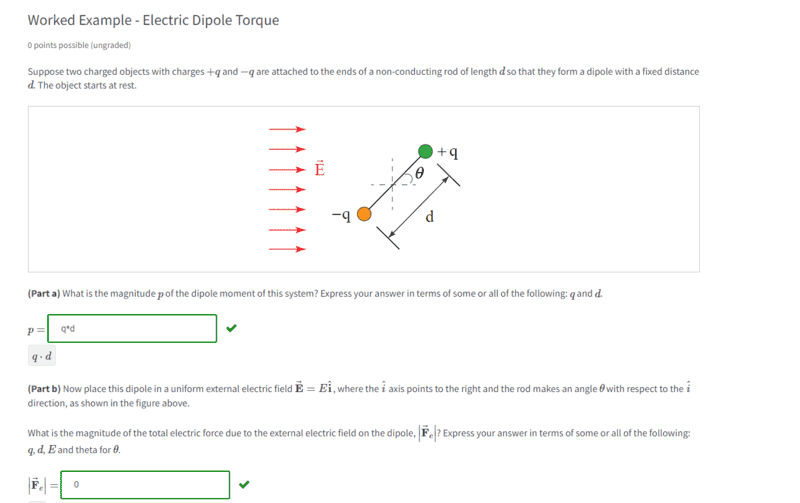I thought it would be: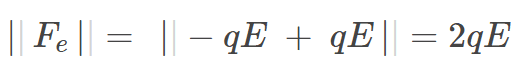If they asked for the total electric force, then I would have said zero because the two electric force vectors cancel.

Many thanks!

#### Attachments

Homework Helper
Gold Member
For this part(b) of this problem, how is the magnitude of the total electric force zero?

I thought it would be:
View attachment 318599
What is the value of ##-qE + qE##? What is the value of ##|-qE + qE|##?

If they asked for the total electric force, then I would have said zero because the two electric force vectors cancel.
Yes, the total electric force vector is the zero vector. So, the magnitude of the total electric force is the magnitude of the zero vector.

If they had asked for the sum of the magnitudes of the two forces, then you would get ##2qE##. But this doesn't have much physical significance. The sum of the magnitudes of two vectors is generally not the same as the magnitude of the sum of the two vectors.

•Callumnc1 and Orodruin
Callumnc1
What is the value of ##-qE + qE##? What is the value of ##|-qE + qE|##?

Yes, the total electric force vector is the zero vector. So, the magnitude of the total electric force is the magnitude of the zero vector.

If they had asked for the sum of the magnitudes of the two forces, then you would get ##2qE##. But this doesn't have much physical significance. The sum of the magnitudes of two vectors is generally not the same as the magnitude of the sum of the two vectors.
Thanks for your help @TSny! The value of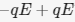is zero. And the value of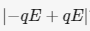is 2qE, correct?

Many thanks!

Staff Emeritus
Homework Helper
Gold Member
•Callumnc1
Callumnc1
Wrong. The difference is taken before the magnitude.
Ok thank you for pointing that out @Orodruin !

Homework Helper
Gold Member
You could also have sorted this out if you kept in mind that force is a vector which has magnitude and direction. Both are needed to specify a vector. In this case, if the force on the dipole were ##2qE##, what would its direction be and why?

•Callumnc1
Callumnc1
You could also have sorted this out if you kept in mind that force is a vector which has magnitude and direction. Both are needed to specify a vector. In this case, if the force on the dipole were ##2qE##, what would its direction be and why?
Thanks for your reply @kuruman ! Is it not impossible for the force on the dipole to be 2qE since the charges are equal and opposite?

Many thanks,
Callum

Homework Helper
Gold Member
It is impossible for the force on the dipole to be 2qE. I was referring to this statement in post #1 where you seem to think that it is 2qE. Did I miss your point? What did you think would be 2qE?

I thought it would be:
View attachment 318599
If they asked for the total electric force, then I would have said zero because the two electric force vectors cancel.

Callumnc1
Thanks for your reply @kuruman! I though the magnitude of the force was 2qE because I though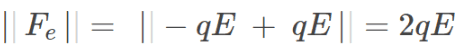. But apparently absolute values bars don't work like that.

I guess 2qE would be the magnitude of the external electric force on each side of the dipole added together, correct?

Many thanks!

Homework Helper
Gold Member
2022 Award
apparently absolute values bars don't work like that.
They could not work like that. The bars mean "take the absolute value of what is between the bars". What is between the bars is -qE+qE, which is zero.
Likewise ##(x+y)^2## is not the same as ##(x^2+y^2)##.

•Callumnc1
Homework Helper
Gold Member
apparently absolute values bars don't work like that.
The bars are like parentheses except, after you calculate what's between them, you strip the negative sign if it's there.

Example
##|5-2|=|3|=3##

##|2-5|=|-3|=3.##

•Callumnc1
Callumnc1
Staff Emeritus
•